# First derivative test facts for kids

Kids Encyclopedia Facts

In calculus, the first derivative test is used to determine local maxima and minima of a function. Furthermore, the first derivative test can be used to determine intervals of increase and intervals of decrease.

## Critical Points (and how to find them)

Critical points are values in the domain of a function where its derivative is 0 or undefined (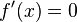$f'(x)=0$ or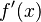$f'(x)$ doesn't exist).

To find the critical points of a function, compute the first derivative of the function and set it equal to zero. Solve for the zeroes of this equation. Similarly, if you have a rational function (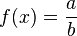$f(x)=\dfrac{a}{b}$, where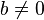$b \neq 0$) find what values will cause the denominator to be equal to 0 because this will make the function undefined, and therefore not differentiable at this point. These are all of your critical numbers.

Plug these numbers into your original function to find the exact coordinate of these critical points.

### Example: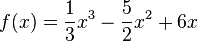$f(x)=\dfrac{1}{3}x^3-\dfrac{5}{2}x^2+6x$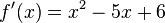$f'(x)=x^2-5x+6$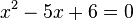$x^2-5x+6=0$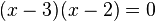$(x-3)(x-2)=0$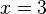$x=3$ and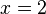$x=2$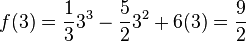$f(3)=\dfrac{1}{3}3^3-\dfrac{5}{2}3^2+6(3)=\dfrac{9}{2}$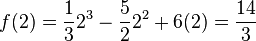$f(2)=\dfrac{1}{3}2^3-\dfrac{5}{2}2^2+6(2)=\dfrac{14}{3}$

So the two critical points are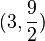$(3,\dfrac{9}{2})$ and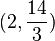$(2,\dfrac{14}{3})$.

## First derivative test

To determine whether these points are local maximas, minimas, or neither, we need to consider the intervals between each of the points. Plug in a value from each interval into the first derivative of the function. If the value is positive, then that is an interval of increase. If the value is negative, then that is an interval of decrease. Finally, if two neighboring intervals change sign then that point is either a local maxima or minima. If the first interval is positive and the second interval negative, then the critical point is a local maxima. If the first interval is negative and the second interval positive, then the critical point is a local minima. If both intervals are positive or both intervals are negative, then the critical point is neither a local maxima or minima.

The first derivative test is used in calculus optimization problems.First derivative test Facts for Kids. Kiddle Encyclopedia.